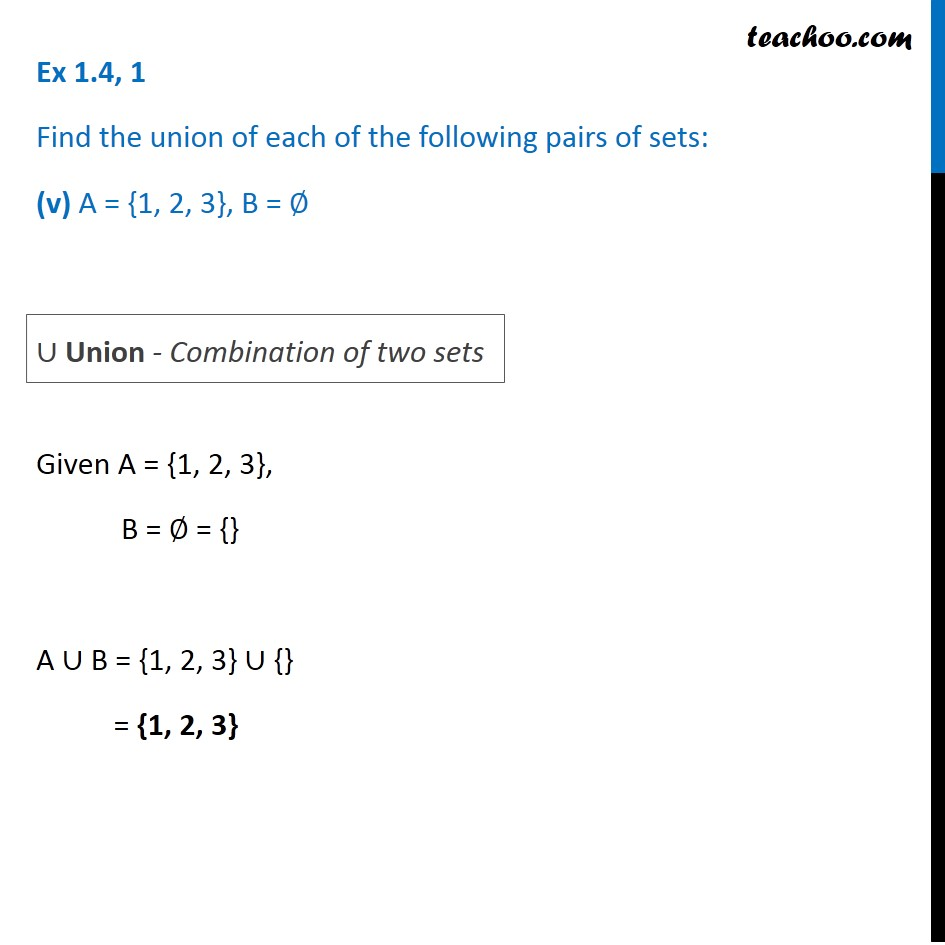1. Chapter 1 Class 11 Sets (Term 1)
2. Serial order wise
3. Ex 1.4

Transcript

Ex 1.4, 1 Find the union of each of the following pairs of sets: (v) A = {1, 2, 3}, B = ∅ Given A = {1, 2, 3}, B = ∅ = {} A ∪ B = {1, 2, 3} ∪ {} = {1, 2, 3} ∪ Union - Combination of two sets